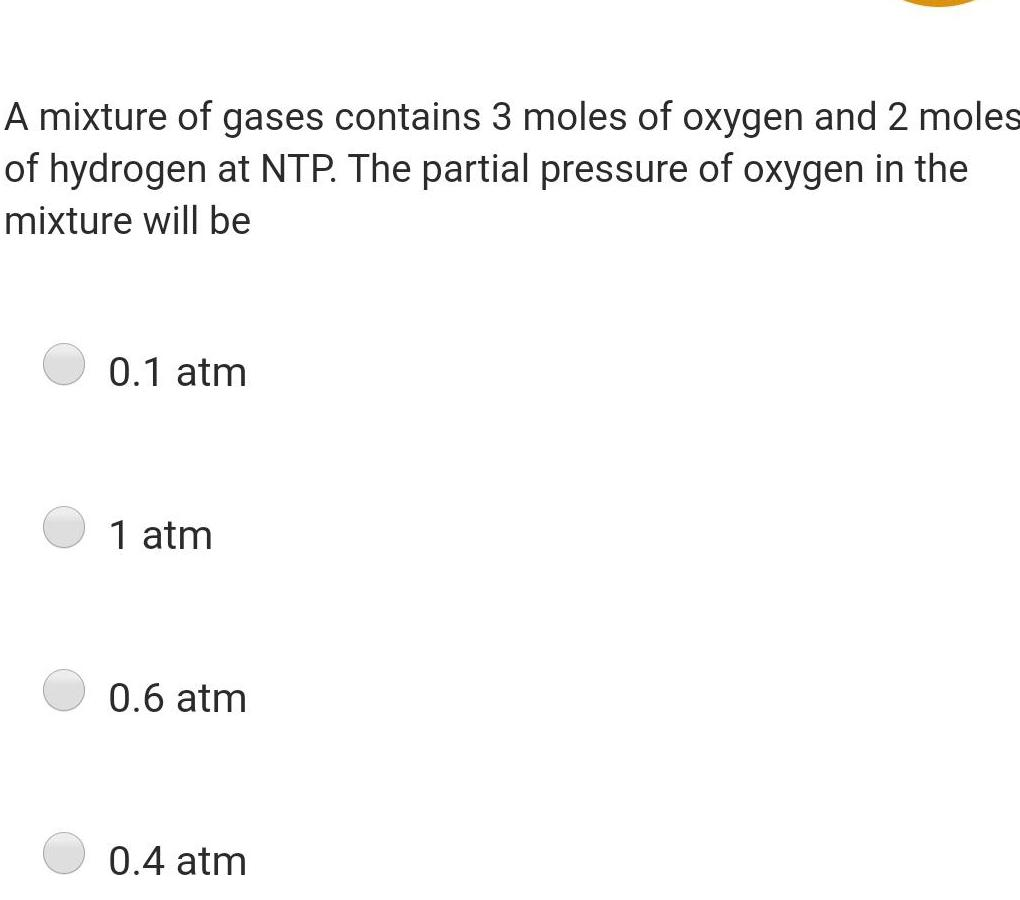Physics
Thermodynamics
A mixture of gases contains 3 moles of oxygen and 2 moles of hydrogen at NTP The partial pressure of oxygen in the mixture will be 0 1 atm 1 atm 0 6 atm 0 4 atm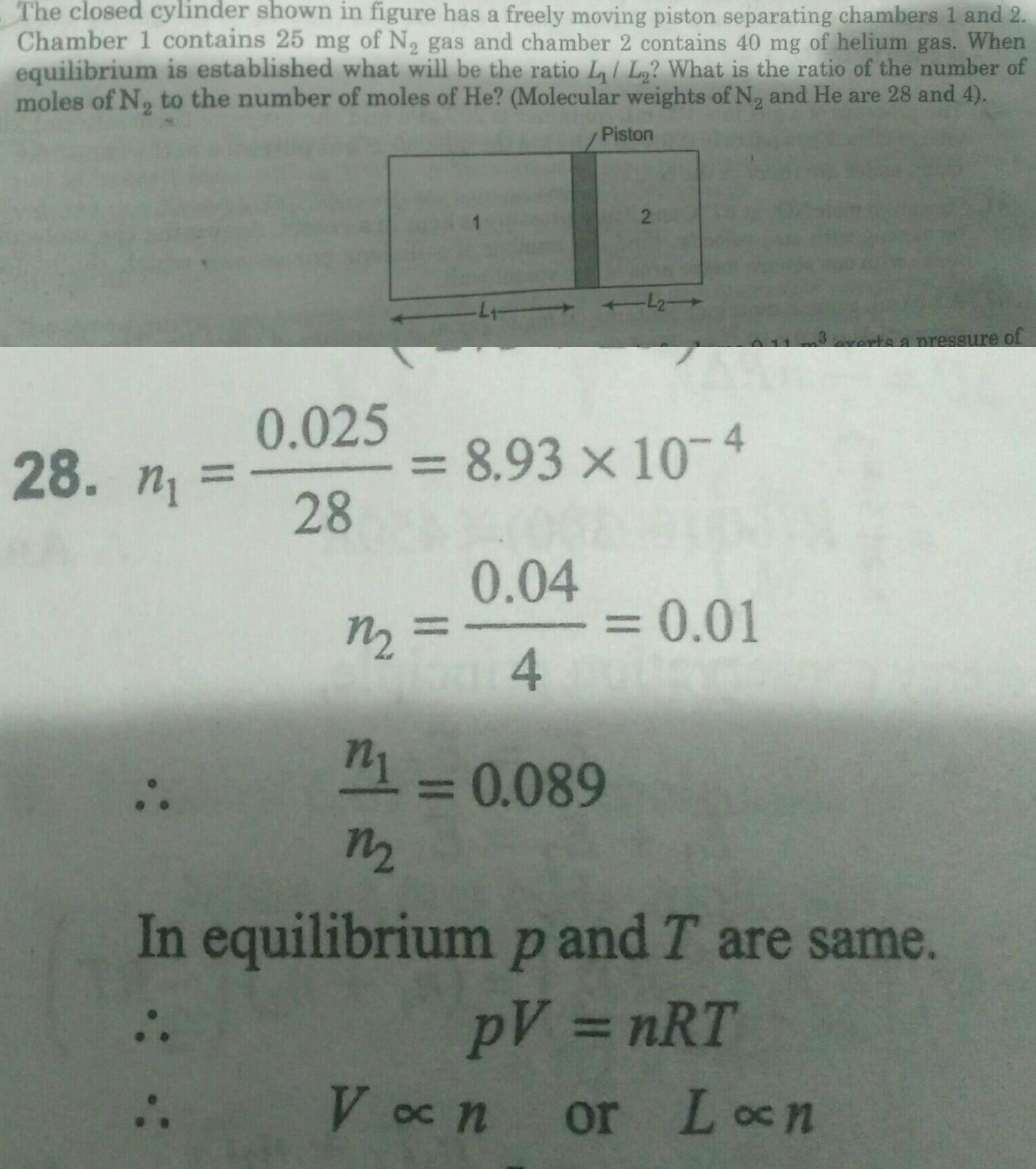Physics
Thermodynamics
The closed cylinder shown in figure has a freely moving piston separating chambers 1 and 2 Chamber 1 contains 25 mg of N gas and chamber 2 contains 40 mg of helium gas When equilibrium is established what will be the ratio L L What is the ratio of the number of moles of N to the number of moles of He Molecular weights of N and He are 28 and 4 28 n 0 025 28 m Piston 0 04 4 8 93 10 4 2 L2 m1 0 089 M 0 01 rts a pressure of In equilibrium p and T are same PV nRT V on or Lon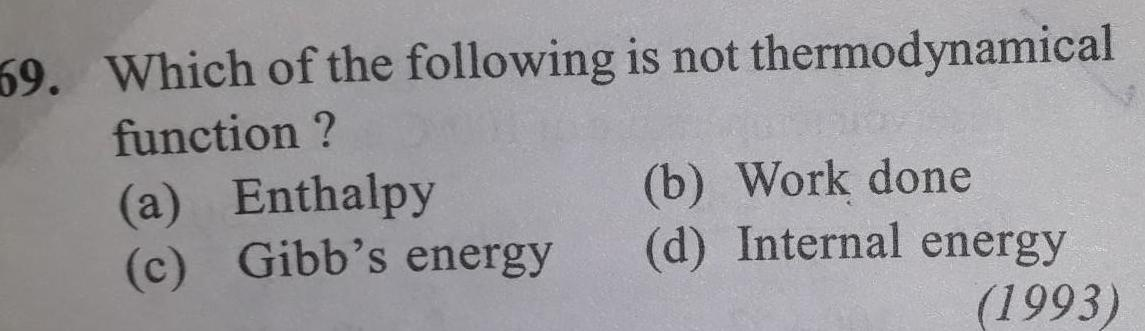Physics
Thermodynamics
59 Which of the following is not thermodynamical function a Enthalpy c Gibb s energy b Work done d Internal energy 1993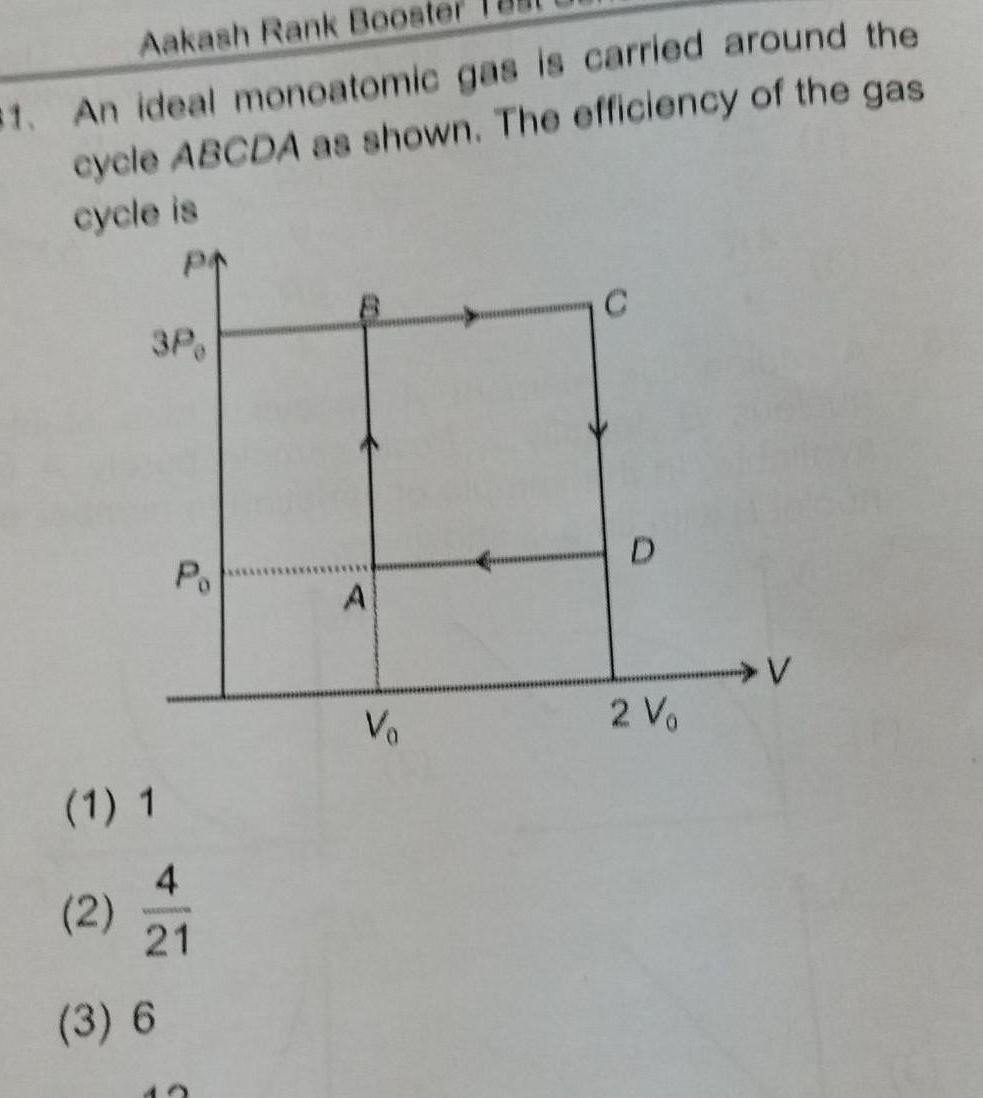Physics
Thermodynamics
Aakash Rank Boost 1 An ideal monoatomic gas is carried around the cycle ABCDA as shown The efficiency of the gas cycle is PA 3P 1 1 2 Po 4 www 21 3 6 A Va C D 2 V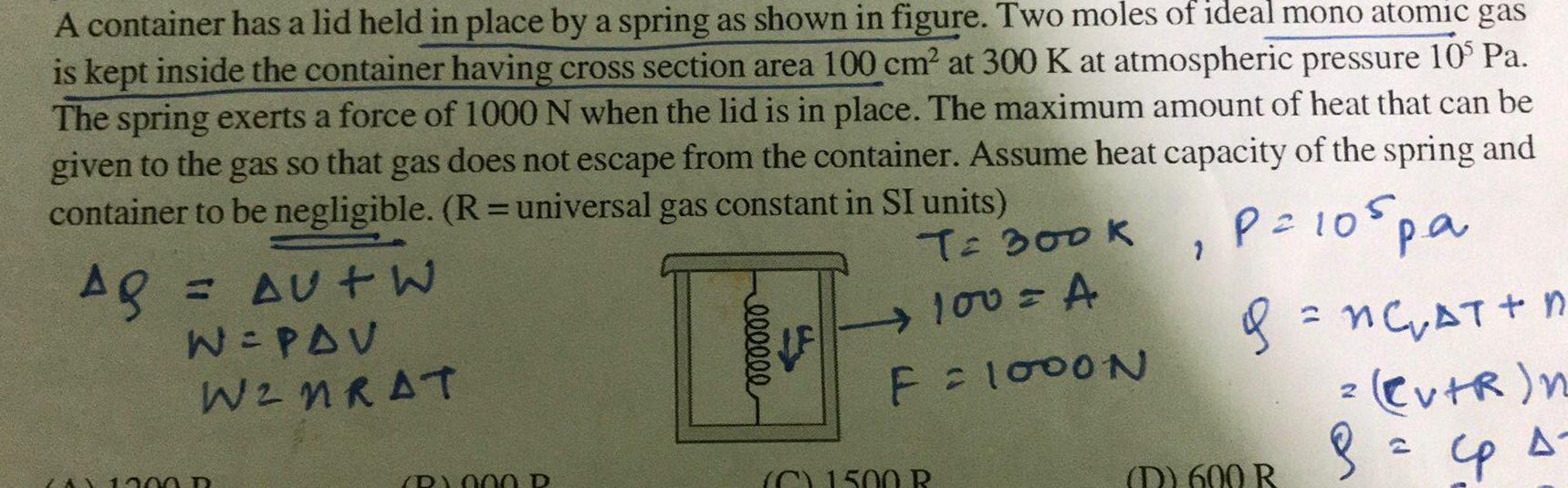Physics
Thermodynamics
A container has a lid held in place by a spring as shown in figure Two moles of ideal mono atomic gas is kept inside the container having cross section area 100 cm at 300 K at atmospheric pressure 10 Pa The spring exerts a force of 1000 N when the lid is in place The maximum amount of heat that can be given to the gas so that gas does not escape from the container Assume heat capacity of the spring and container to be negligible R universal gas constant in SI units T 300K Ag P 105 pa 100 A F 1000N AU W W PAV W2NRAT ZAX 1000 P B 000 P I eeeeee C1500 B g nC AT n Cv R n 2 8 Cp s D 600 R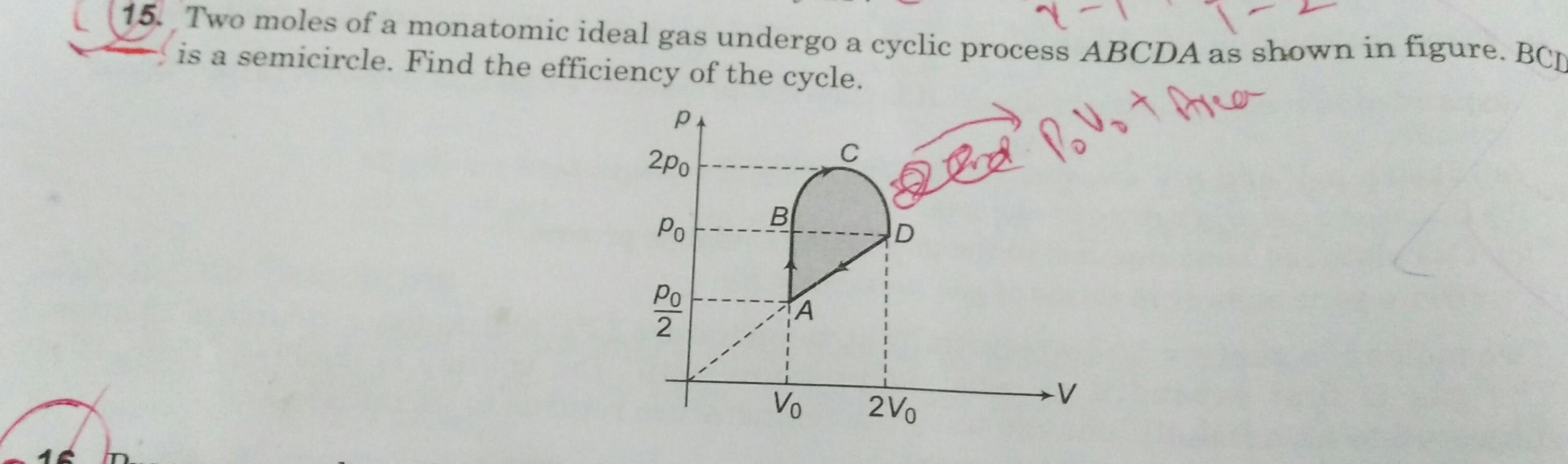Physics
Thermodynamics
16 15 Two moles of a monatomic ideal gas undergo a cyclic process ABCDA as shown in figure BCD is a semicircle Find the efficiency of the cycle PA Id Povot Area 2po Po Po 2 I I 1 B A Vo C 2V V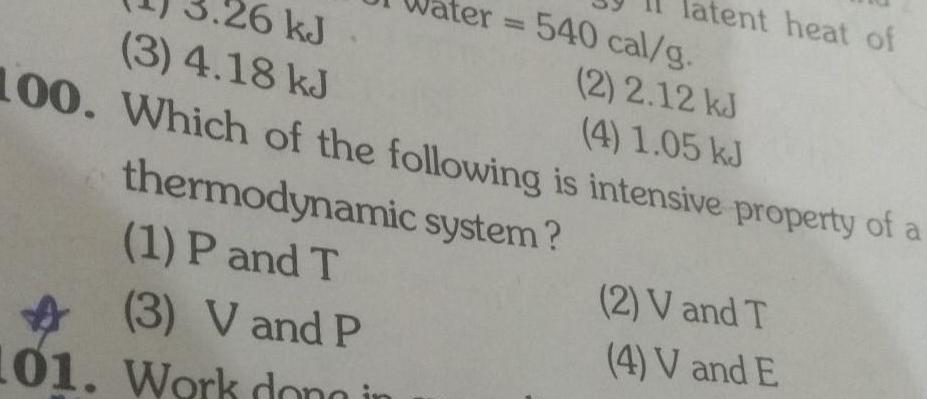Physics
Thermodynamics
26 kJ 2 2 12 kJ 3 4 18 kJ 4 1 05 kJ 100 Which of the following is intensive property of a 540 cal g thermodynamic system 1 P and T 3 V and P 101 Work dono in tent heat of 2 V and T 4 V and E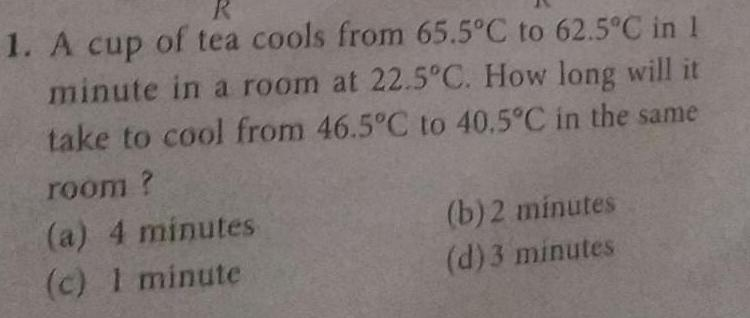Physics
Thermodynamics
1 A cup of tea cools from 65 5 C to 62 5 C in 1 minute in a room at 22 5 C How long will it take to cool from 46 5 C to 40 5 C in the same room a 4 minutes c 1 minute b 2 minutes d 3 minutes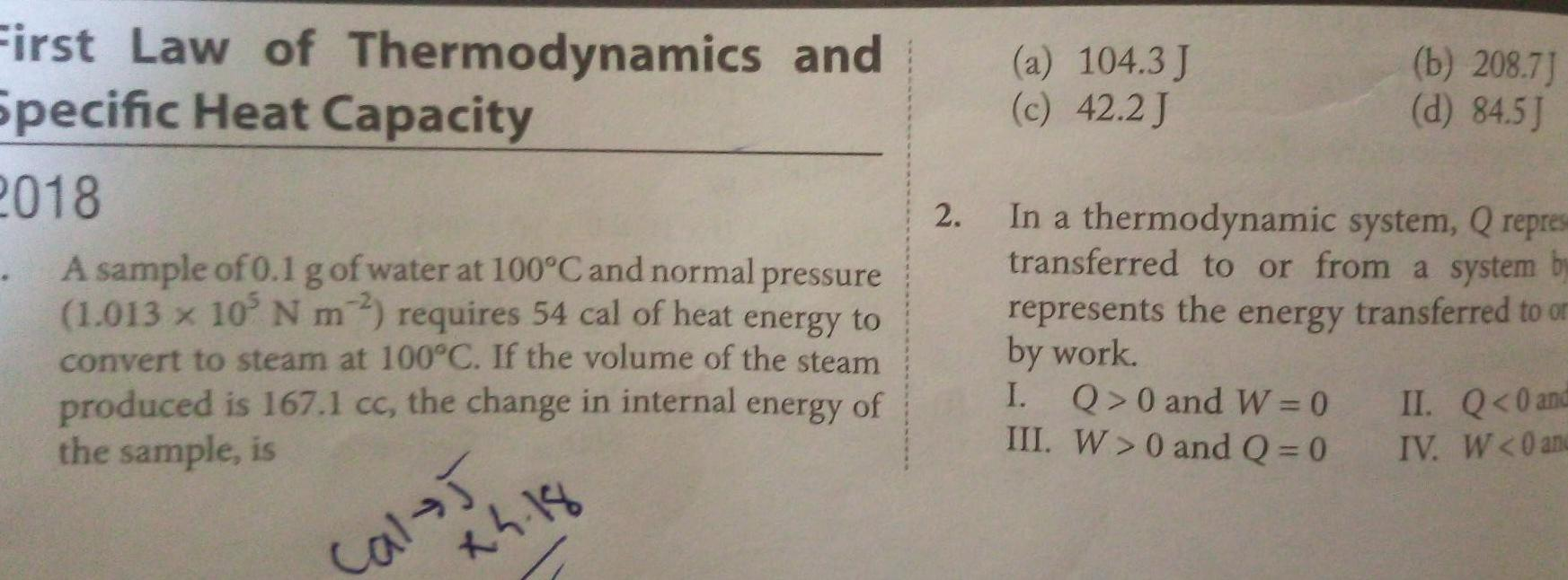Physics
Thermodynamics
First Law of Thermodynamics and Specific Heat Capacity 2018 A sample of 0 1 g of water at 100 C and normal pressure 1 013 x 105 N m2 requires 54 cal of heat energy to convert to steam at 100 C If the volume of the steam produced is 167 1 cc the change in internal energy of the sample is 2 18 Cal 5 2 a 104 3 J c 42 2 J b 208 7 d 84 5J In a thermodynamic system Q repres transferred to or from a system by represents the energy transferred to or by work I Q 0 and W 0 III W 0 and Q 0 II Q 0 and IV W 0 and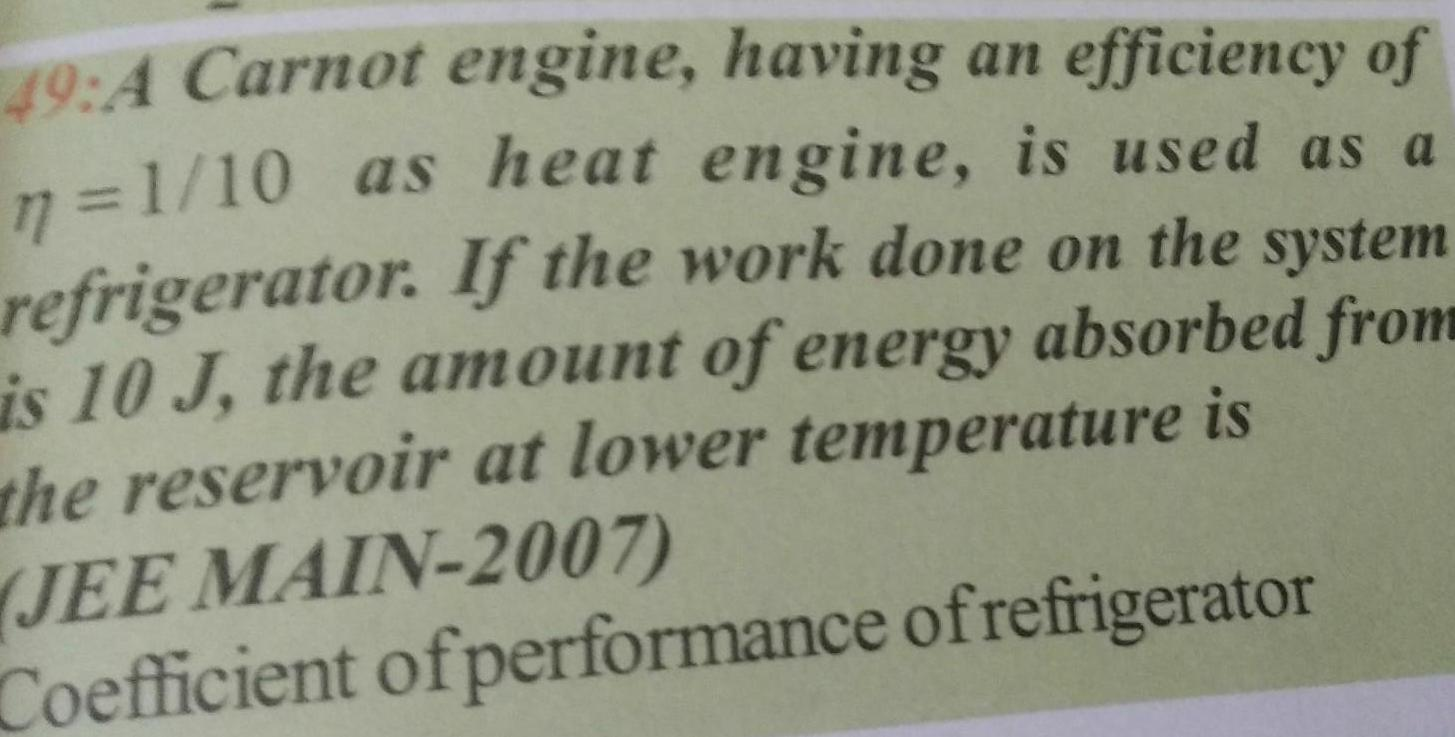Physics
Thermodynamics
49 A Carnot engine having an efficiency of n 1 10 as heat engine is used as a refrigerator If the work done on the system is 10 J the amount of energy absorbed from the reservoir at lower temperature is JEE MAIN 2007 Coefficient of performance of refrigerator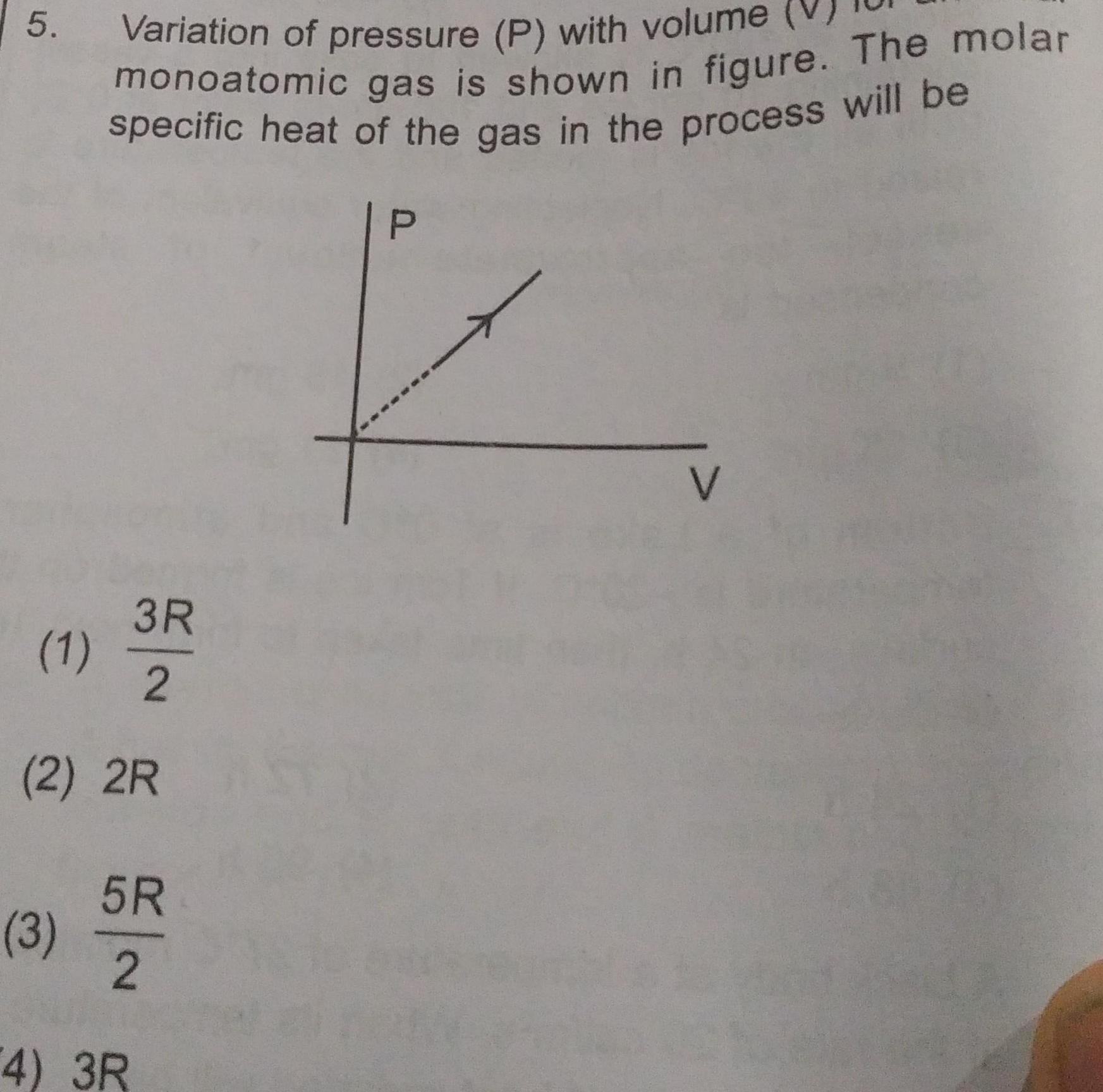Physics
Thermodynamics
5 3R 2 2 2R 1 Variation of pressure P with volume monoatomic gas is shown in figure The molar specific heat of the gas in the process will be 3 5R 2 4 3R P V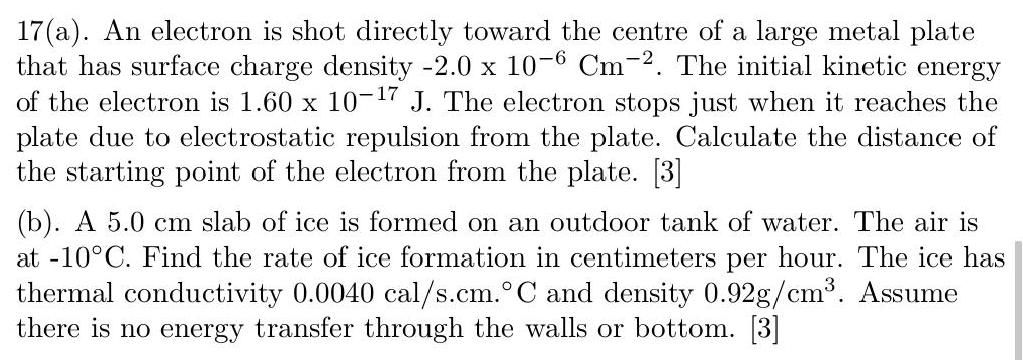Physics
Thermodynamics
17 a An electron is shot directly toward the centre of a large metal plate that has surface charge density 2 0 x 10 6 Cm 2 The initial kinetic energy of the electron is 1 60 x 10 7 J The electron stops just when it reaches the plate due to electrostatic repulsion from the plate Calculate the distance of the starting point of the electron from the plate 3 b A 5 0 cm slab of ice is formed on an outdoor tank of water The air is at 10 C Find the rate of ice formation in centimeters per hour The ice has thermal conductivity 0 0040 cal s cm C and density 0 92g cm Assume there is no energy transfer through the walls or bottom 3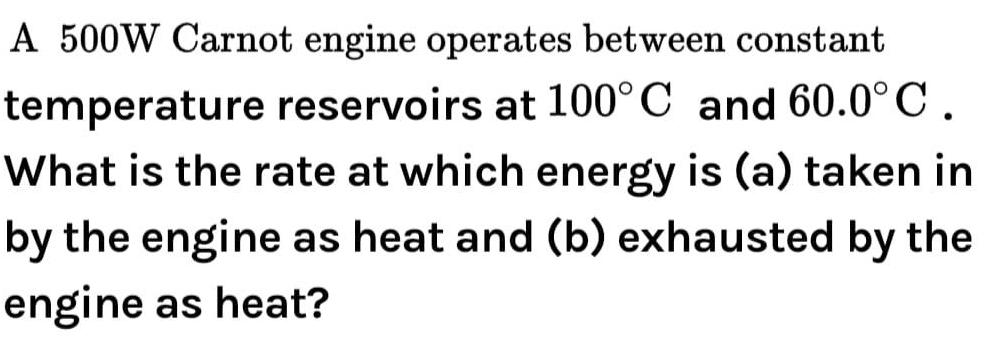Physics
Thermodynamics
A 500W Carnot engine operates between constant temperature reservoirs at 100 C and 60 0 C What is the rate at which energy is a taken in by the engine as heat and b exhausted by the engine as heat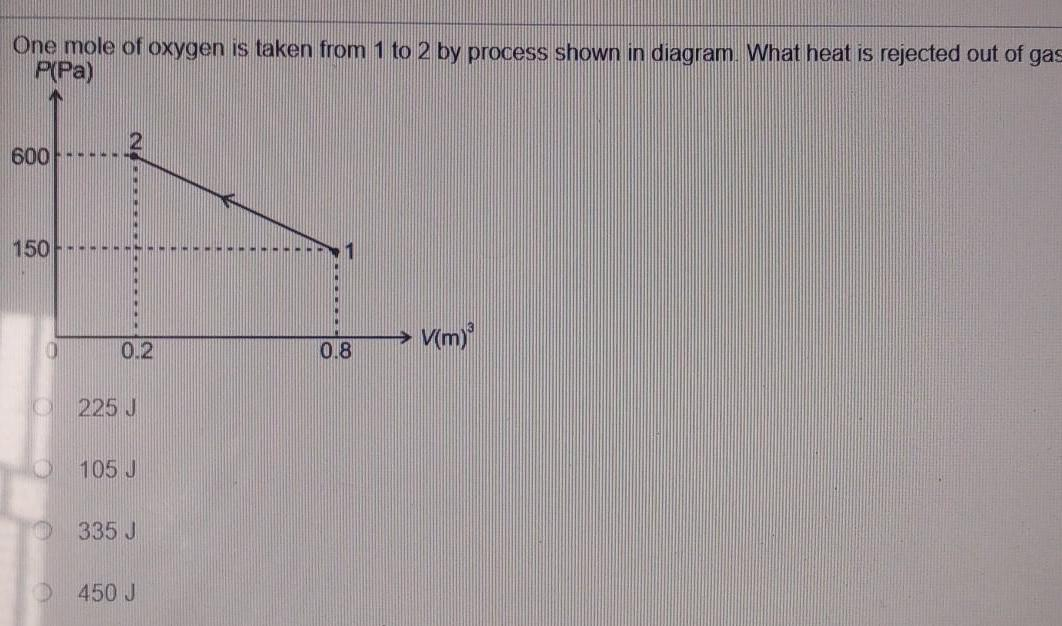Physics
Thermodynamics
One mole of oxygen is taken from 1 to 2 by process shown in diagram What heat is rejected out of gas P Pa 600 150 0 ww 0 2 225 J 105 J 335 J 450 J 1 0 8 V m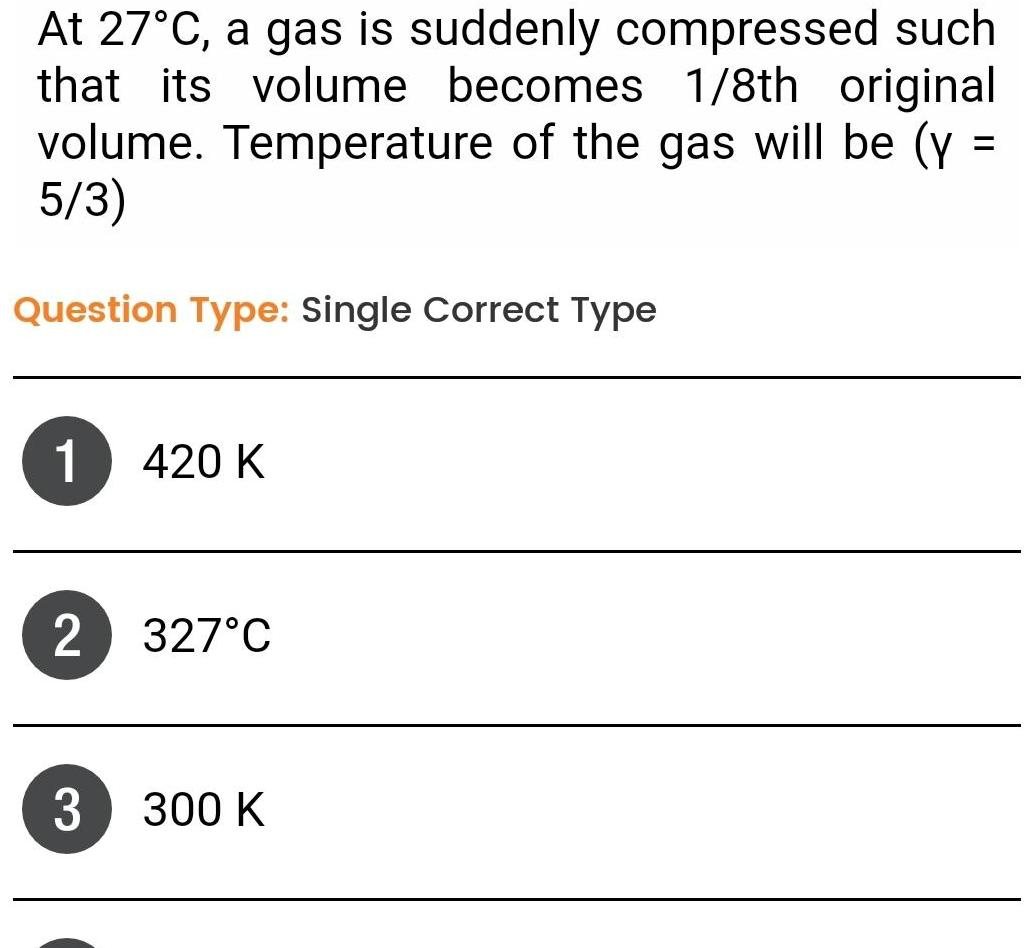Physics
Thermodynamics
At 27 C a gas is suddenly compressed such that its volume becomes 1 8th original volume Temperature of the gas will be y 5 3 Question Type Single Correct Type 1 420 K 2 327 C 3 300 K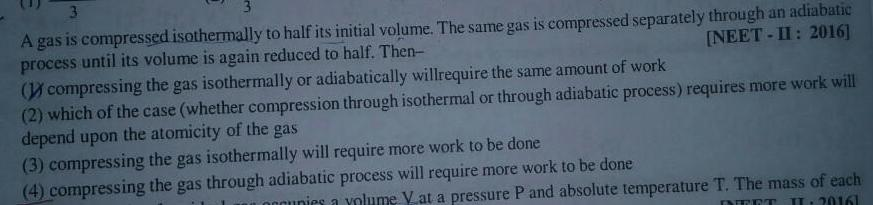Physics
Thermodynamics
3 3 A gas is compressed isothermally to half its initial volume The same gas is compressed separately through an adiabatic process until its volume is again reduced to half Then NEET II 2016 compressing the gas isothermally or adiabatically willrequire the same amount of work 2 which of the case whether compression through isothermal or through adiabatic process requires more work will depend upon the atomicity of the gas 3 compressing the gas isothermally will require more work to be done 4 compressing the gas through adiabatic process will require more work to be done Loccupies a volume V at a pressure P and absolute temperature T The mass of each INEET IL 20161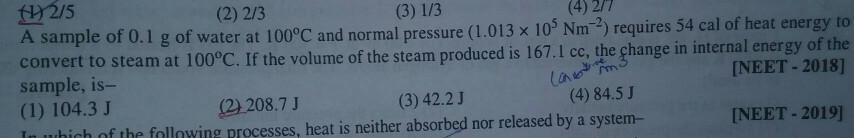Physics
Thermodynamics
1 2 5 2 2 3 3 1 3 4 2 7 A sample of 0 1 g of water at 100 C and normal pressure 1 013 x 105 Nm2 requires 54 cal of heat energy to convert to steam at 100 C If the volume of the steam produced is 167 1 cc the change in internal energy of the sample is NEET 2018 1 104 3 J 2 208 7 J 3 4 84 5 J 3 42 2 J In which of the following processes heat is neither absorbed nor released by a system NEET 2019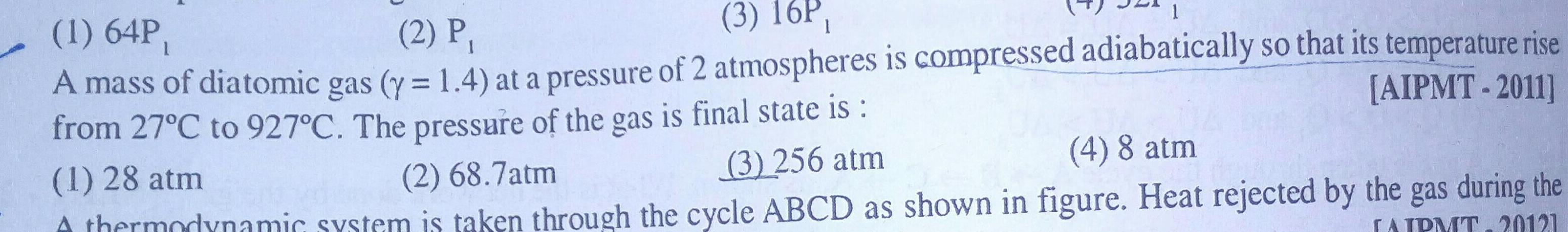Physics
Thermodynamics
1 1 64P 3 16P 2 P AIPMT 2011 A mass of diatomic gas y 1 4 at a pressure of 2 atmospheres is compressed adiabatically so that its temperature rise from 27 C to 927 C The pressure of the gas is final state is 3 256 atm 1 28 atm 2 68 7atm 4 8 atm A thermodynamic system is taken through the cycle ABCD as shown in figure Heat rejected by the gas during the LAIPMT20121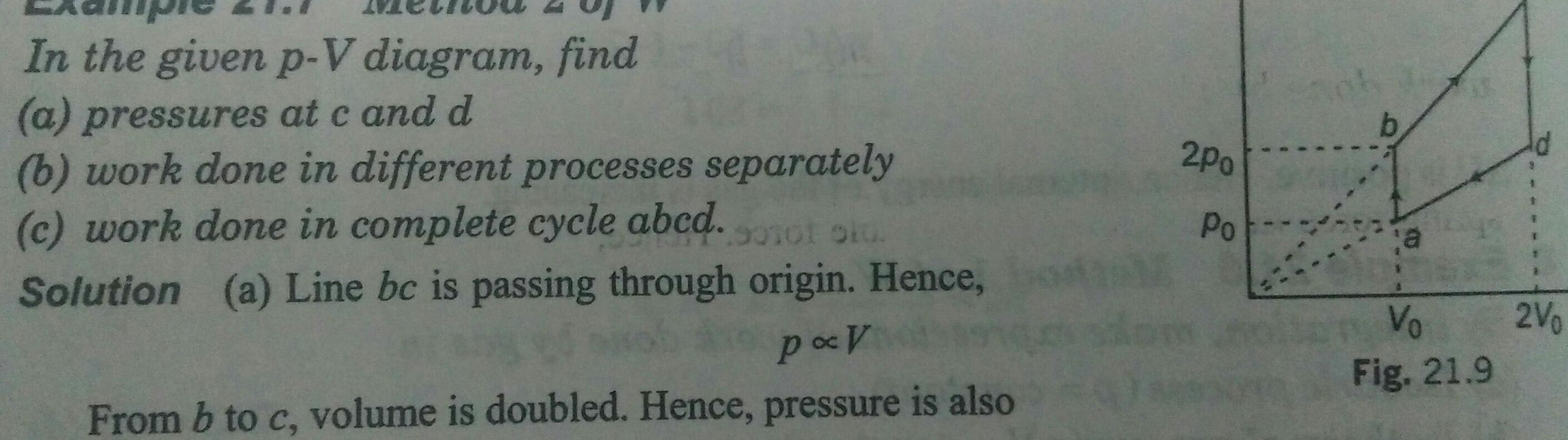Physics
Thermodynamics
In the given p V diagram find a pressures at c and d b work done in different processes separately c work done in complete cycle abcd 90101 910 Solution a Line bc is passing through origin Hence po V From b to c volume is doubled Hence pressure is also 2po Po Vo Fig 21 9 2V0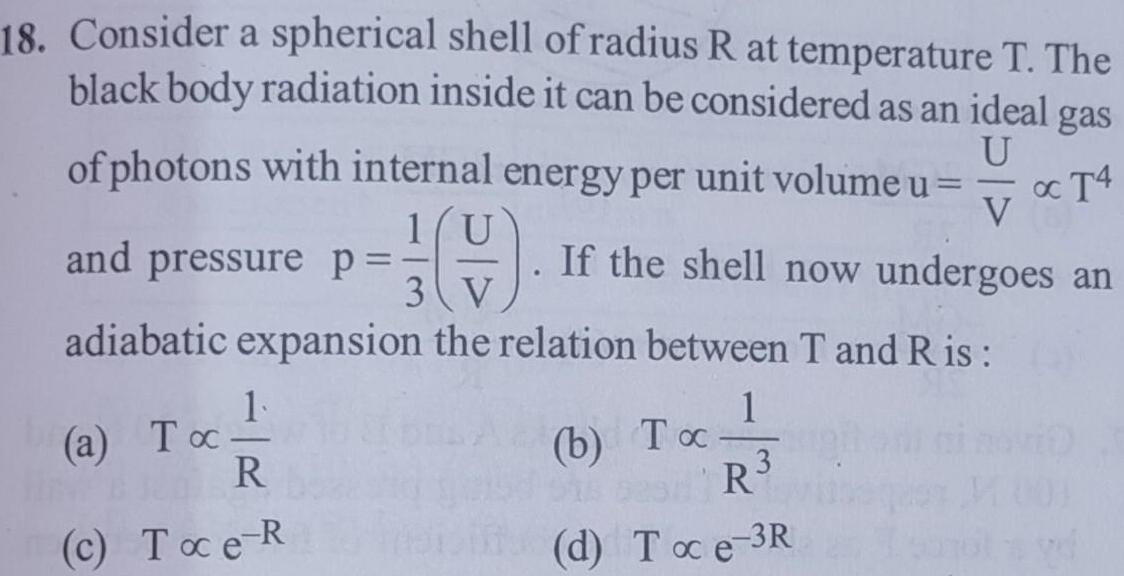Physics
Thermodynamics
18 Consider a spherical shell of radius R at temperature T The black body radiation inside it can be considered as an ideal gas of photons with internal energy per unit volume u U o T4 1 U V If the shell now undergoes an and pressure p 3 V adiabatic expansion the relation between T and Ris 1 1 a Toc R c Toce R b To R d Toc e 3R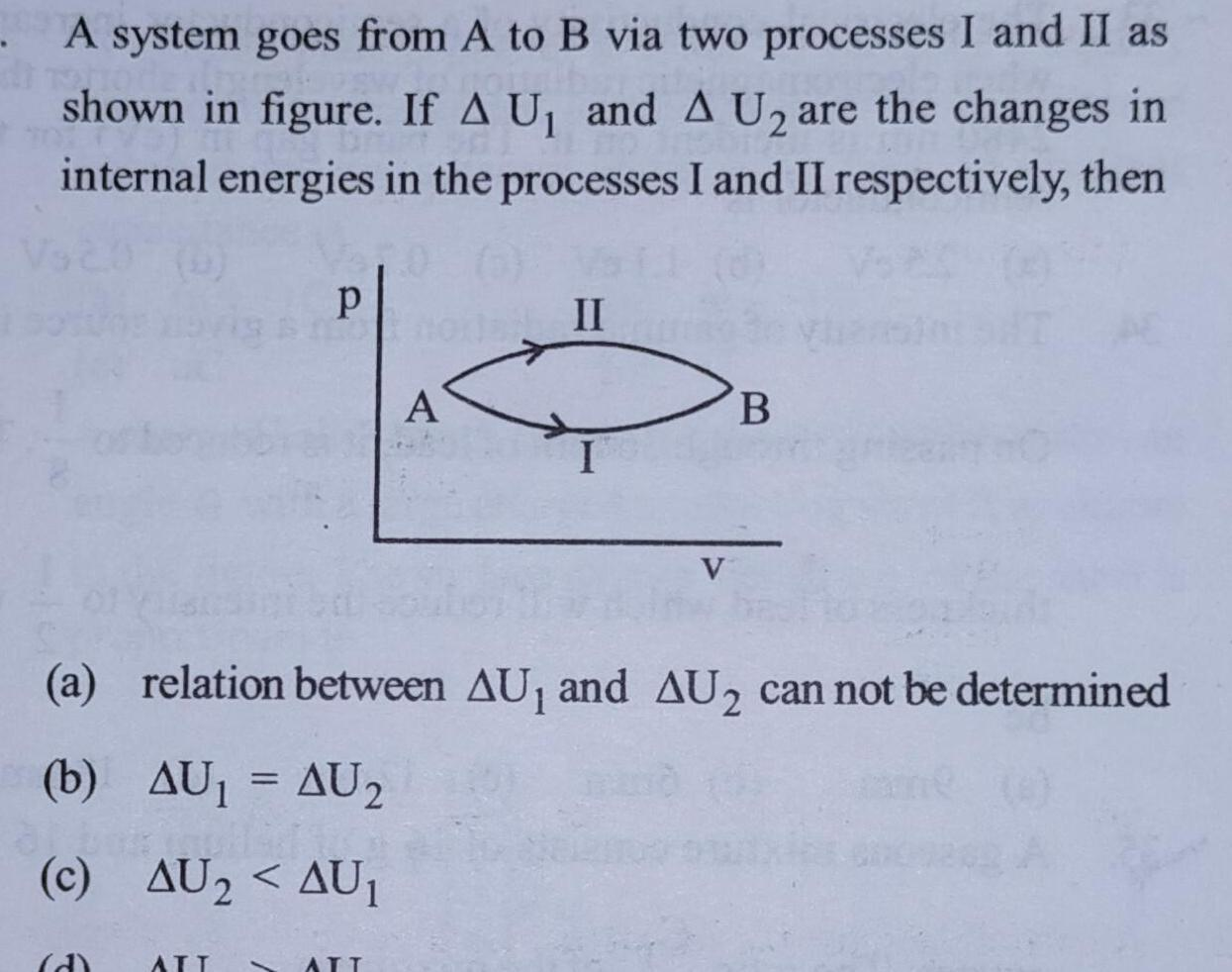Physics
Thermodynamics
A system goes from A to B via two processes I and II as shown in figure If A U and A U are the changes in internal energies in the processes I and II respectively then p 8 none II A a relation between AU and AU can not be determined b AU AU2 c AU AU ATT 1 ATT B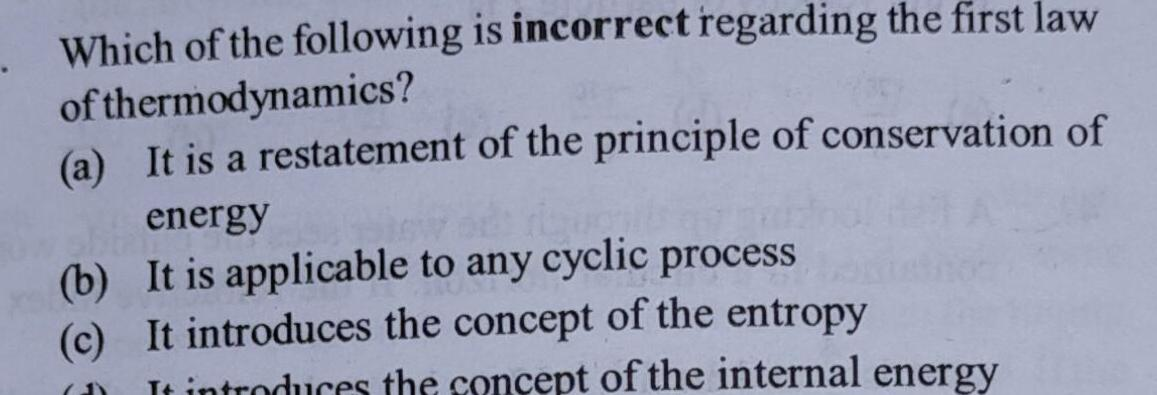Physics
Thermodynamics
Which of the following is incorrect regarding the first law of thermodynamics a It is a restatement of the principle of conservation of energy b It is applicable to any cyclic process c It introduces the concept of the entropy D It introduces the concept of the internal energy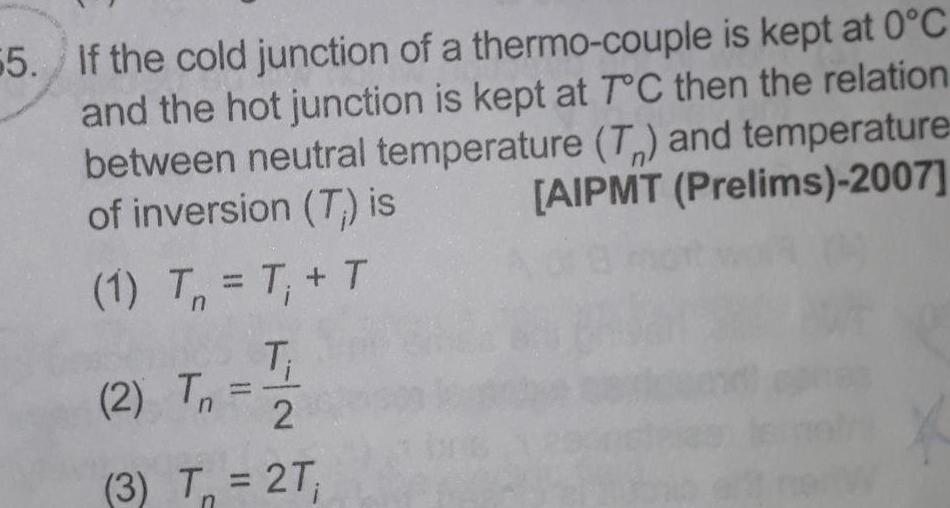Physics
Thermodynamics
55 If the cold junction of a thermo couple is kept at 0 C and the hot junction is kept at T C then the relation between neutral temperature T and temperature of inversion T is AIPMT Prelims 2007 1 T T T T 2 2 T Tn 3 T 2T n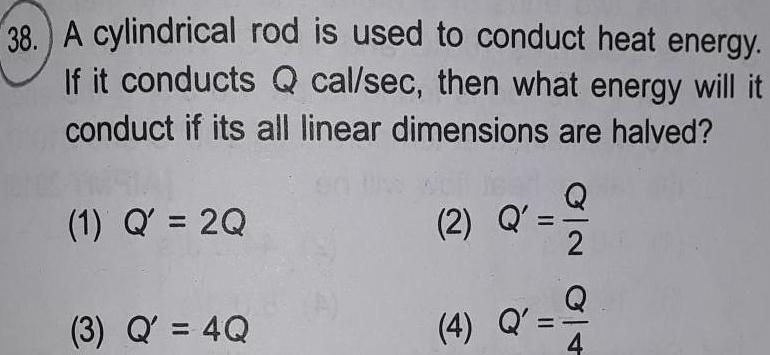Physics
Thermodynamics
38 A cylindrical rod is used to conduct heat energy If it conducts Q cal sec then what energy will it conduct if its all linear dimensions are halved 1 Q 2Q 3 Q 4Q 2 Q 4 Q ON OT 2 Q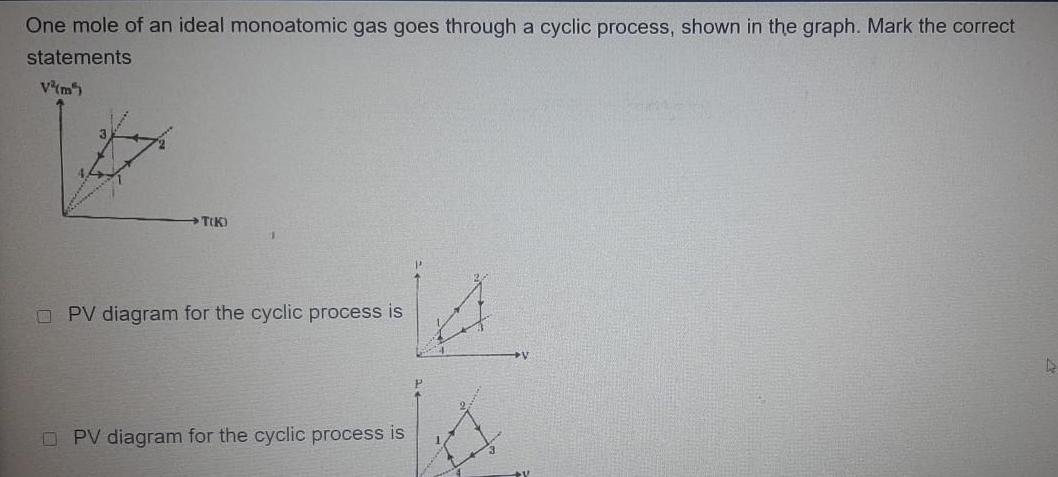Physics
Thermodynamics
One mole of an ideal monoatomic gas goes through a cyclic process shown in the graph Mark the correct statements V m5 T K PV diagram for the cyclic process is PV diagram for the cyclic process is D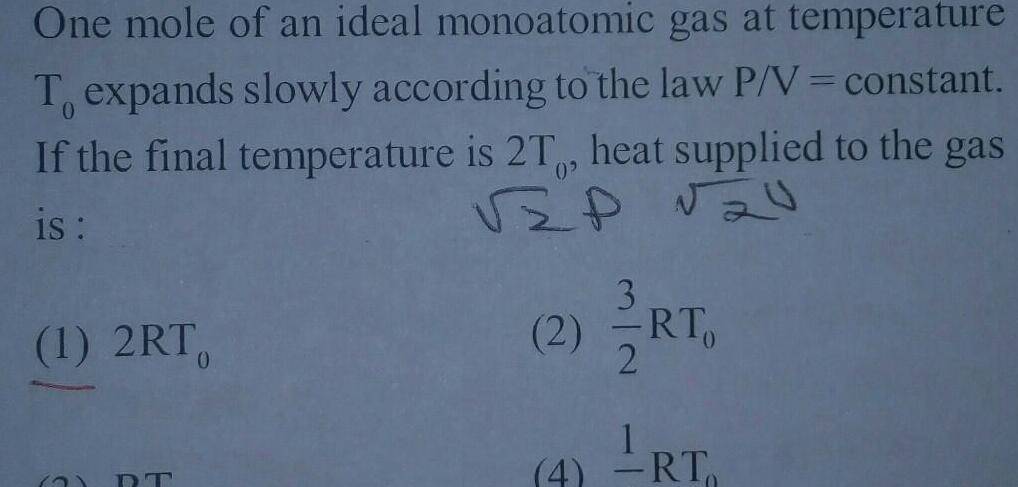Physics
Thermodynamics
One mole of an ideal monoatomic gas at temperature T expands slowly according to the law P V constant If the final temperature is 2T heat supplied to the gas is VZP 20 1 2RT 2 3 2 RT 2 LRT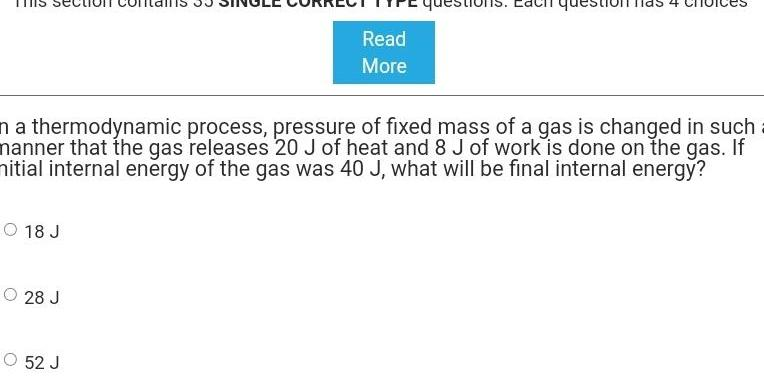Physics
Thermodynamics
O 18 J O 28 J Read More n a thermodynamic process pressure of fixed mass of a gas is changed in such a manner that the gas releases 20 J of heat and 8 J of work is done on the gas If nitial internal energy of the gas was 40 J what will be final internal energy O 52 J estio question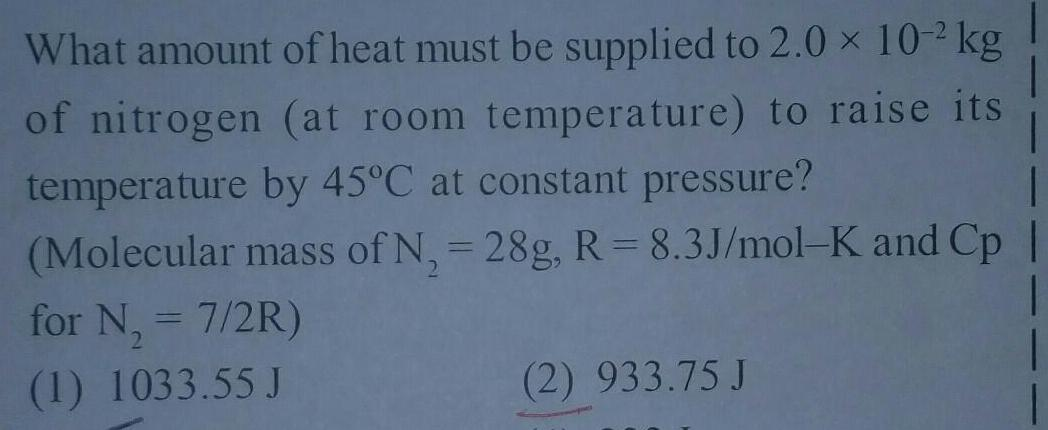Physics
Thermodynamics
What amount of heat must be supplied to 2 0 10 2 kg of nitrogen at room temperature to raise its temperature by 45 C at constant pressure Molecular mass of N 28g R 8 3J mol K and Cp for N 7 2R 2 1 1033 55 J 2 933 75 J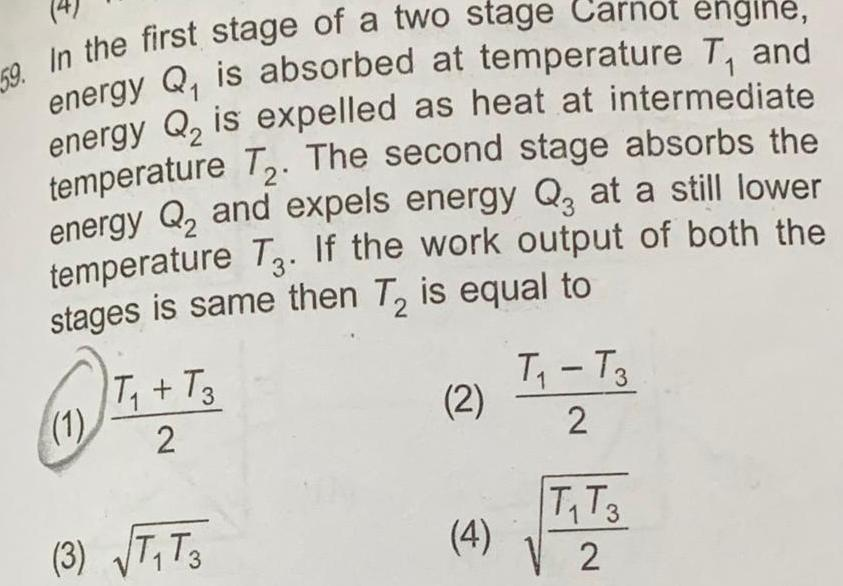Physics
Thermodynamics
59 In the first stage of a two stage Carnot engine energy Q is absorbed at temperature T and energy Q is expelled as heat at intermediate temperature T The second stage absorbs the energy Q and expels energy Q at a still lower temperature T3 If the work output of both the stages is same then T is equal to 2 1 T T3 2 3 T T3 2 4 T T3 2 T T3 2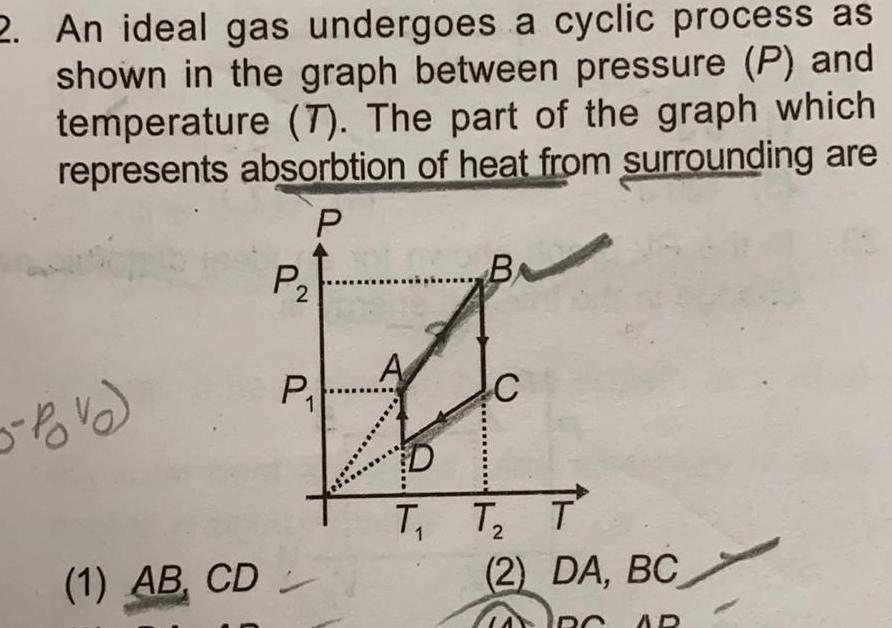Physics
Thermodynamics
2 An ideal gas undergoes a cyclic process as shown in the graph between pressure P and temperature 7 The part of the graph which represents absorbtion of heat from surrounding are P 5 Povo 1 AB CD P P A B 1 2 T T T 2 DA BC AD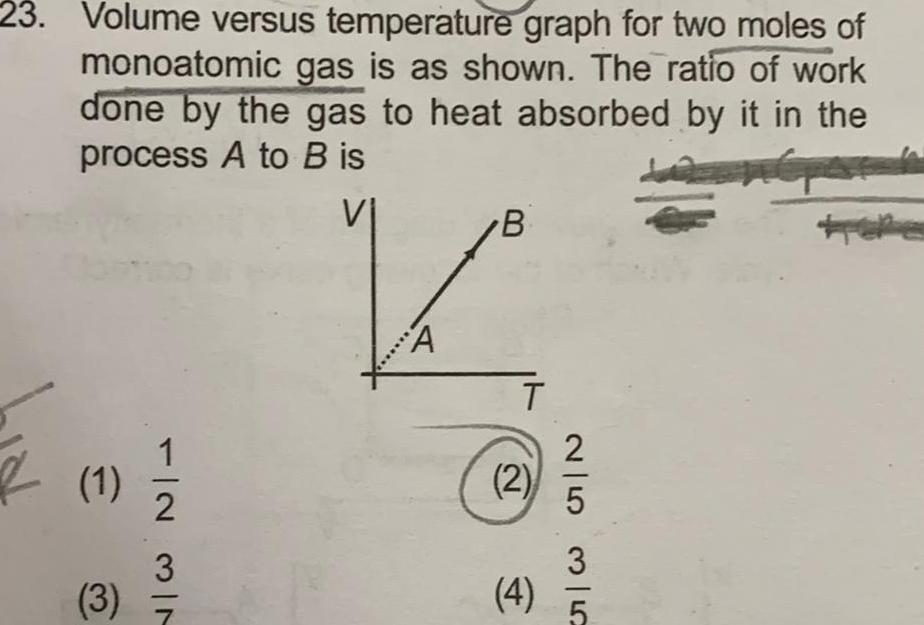Physics
Thermodynamics
23 Volume versus temperature graph for two moles of monoatomic gas is as shown The ratio of work done by the gas to heat absorbed by it in the process A to B is VI 1 3 2 3 7 A B T 2 4 25 352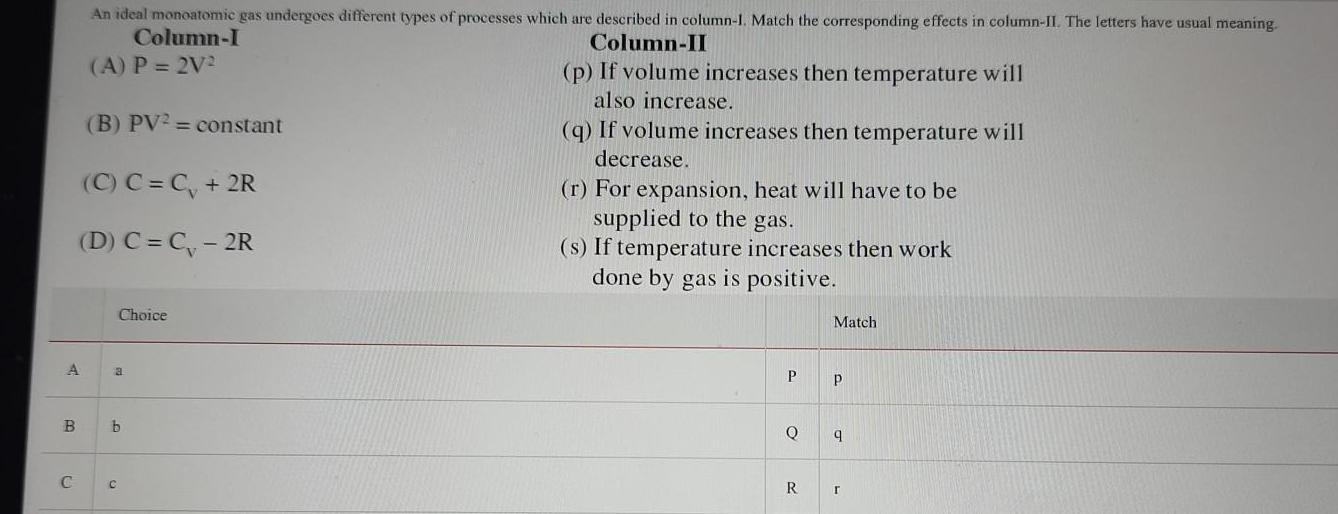Physics
Thermodynamics
A B C An ideal monoatomic gas undergoes different types of processes which are described in column 1 Match the corresponding effects in column II The letters have usual meaning Column I Column II A P 2V p If volume increases then temperature will also increase q If volume increases then temperature will decrease B PV constant C C C 2R D C C 2R Choice a b r For expansion heat will have to be supplied to the gas s If temperature increases then work done by gas is positive P Q R Match p 9 r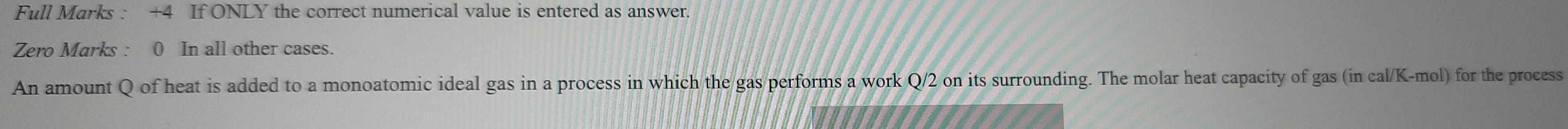Physics
Thermodynamics
Full Marks 4 If ONLY the correct numerical value is entered as answer Zero Marks 0 In all other cases An amount Q of heat is added to a monoatomic ideal gas in a process in which the gas performs a work Q 2 on its surrounding The molar heat capacity of gas in cal K mol for the process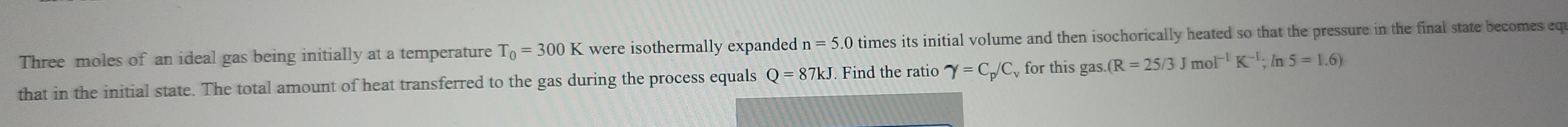Physics
Thermodynamics
Three moles of an ideal gas being initially at a temperature To 300 K were isothermally expanded n 5 0 times its initial volume and then isochorically heated so that the pressure in the final state becomes equ that in the initial state The total amount of heat transferred to the gas during the process equals Q 87kJ Find the ratio Y C C for this gas R 25 3 J mol K In 5 1 6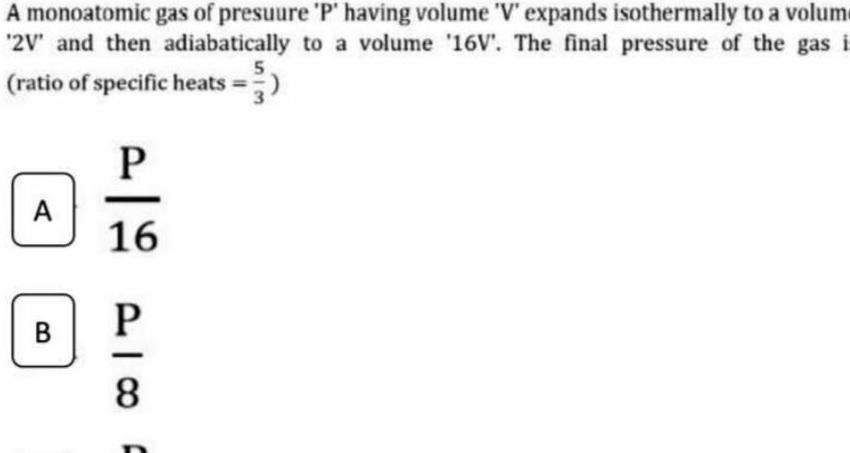Physics
Thermodynamics
A monoatomic gas of presuure P having volume V expands isothermally to a volum 2V and then adiabatically to a volume 16V The final pressure of the gas i 5 ratio of specific heats A B P 16 P 8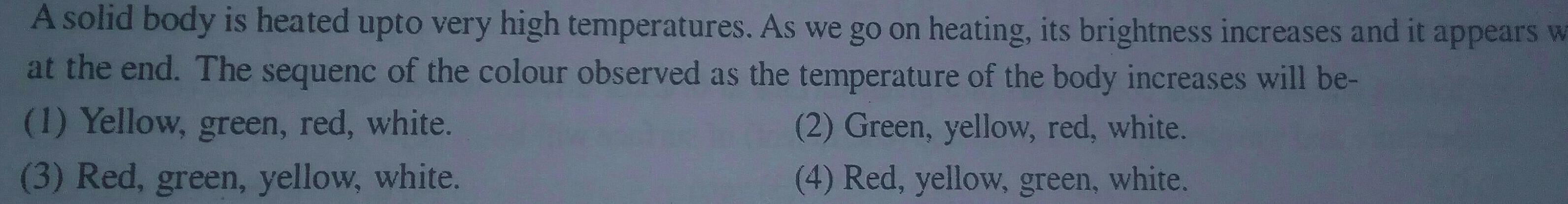Physics
Thermodynamics
A solid body is heated upto very high temperatures As we go on heating its brightness increases and it appears w at the end The sequenc of the colour observed as the temperature of the body increases will be 2 Green yellow red white 1 Yellow green red white 3 Red green yellow white 4 Red yellow green white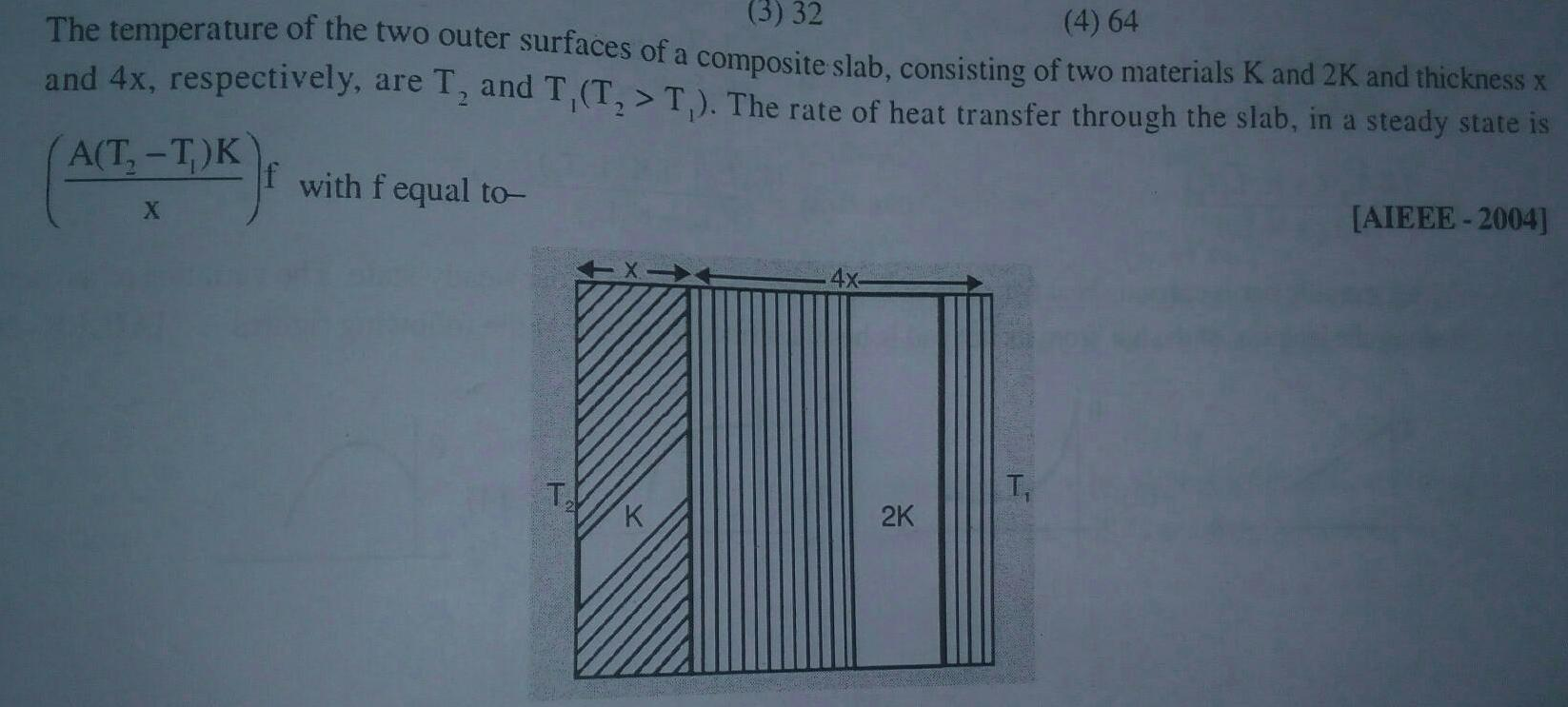Physics
Thermodynamics
3 32 4 64 The temperature of the two outer surfaces of a composite slab consisting of two materials K and 2K and thickness x and 4x respectively are T and T T T The rate of heat transfer through the slab in a steady state is A T T K X with f equal to T K 2K AIEEE 2004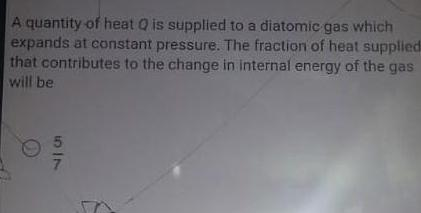Physics
Thermodynamics
A quantity of heat Q is supplied to a diatomic gas which expands at constant pressure The fraction of heat supplied that contributes to the change in internal energy of the gas will be 517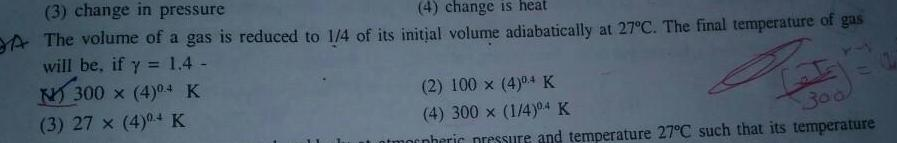Physics
Thermodynamics
3 change in pressure 4 change is heat A The volume of a gas is reduced to 1 4 of its initial volume adiabatically at 27 C The final temperature of gas will be if y 1 4 N300 4 0 4 K 3 27 x 4 0 K Pry 300 2 100 x 4 0 4 K 4 300 x 1 4 0 4 K morpheric pressure and temperature 27 C such that its temperature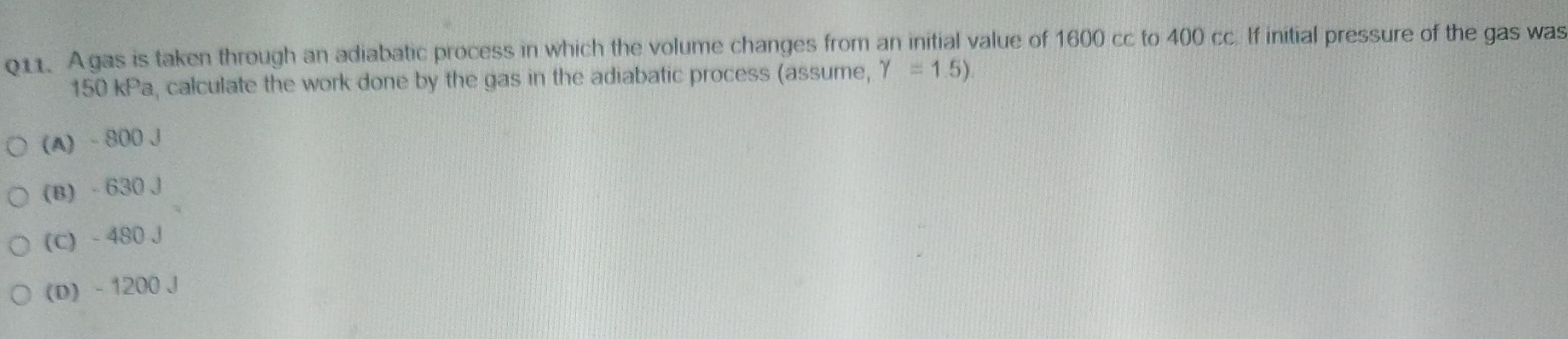Physics
Thermodynamics
Q11 Agas is taken through an adiabatic process in which the volume changes from an initial value of 1600 cc to 400 cc If initial pressure of the gas was 150 kPa calculate the work done by the gas in the adiabatic process assume Y 1 5 O A 800 J O B 630 J O C 480 J O D 1200 J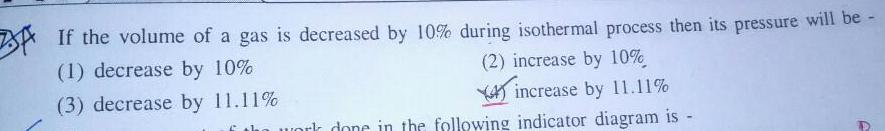Physics
Thermodynamics
BA If the volume of a gas is decreased by 10 during isothermal process then its pressure will be 1 decrease by 10 2 increase by 10 3 decrease by 11 11 increase by 11 11 work done in the following indicator diagram is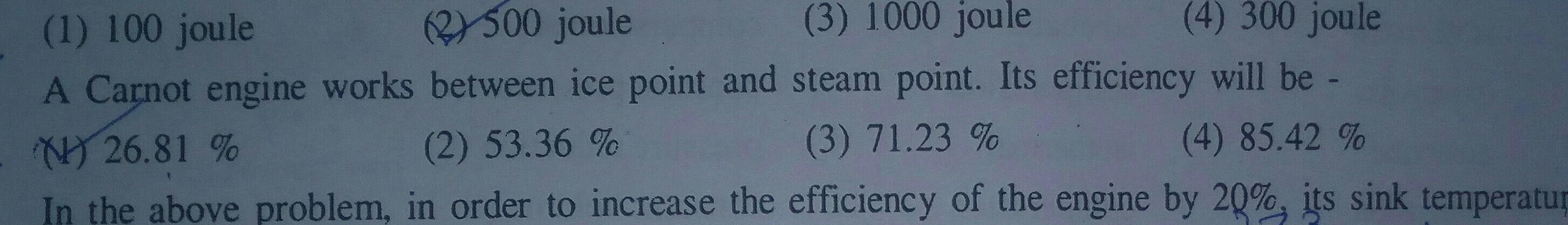Physics
Thermodynamics
1 100 joule 2 500 joule 3 1000 joule 4 300 joule A Carnot engine works between ice point and steam point Its efficiency will be 26 81 2 53 36 3 71 23 4 85 42 In the above problem in order to increase the efficiency of the engine by 20 its sink temperatur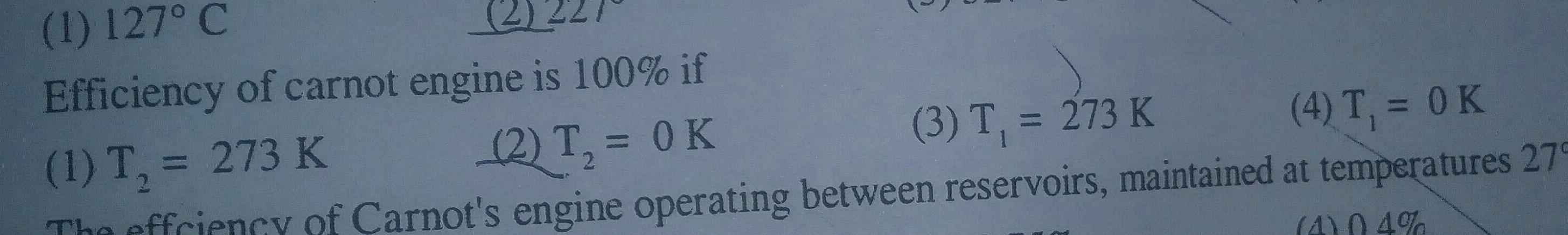Physics
Thermodynamics
1 127 C Efficiency of carnot engine is 100 if 1 T 273 K 2 T 0 K 3 T 273 K 2 The effriency of Carnot s engine operating between reservoirs maintained 4 T 0 K at temperatures 279 4 04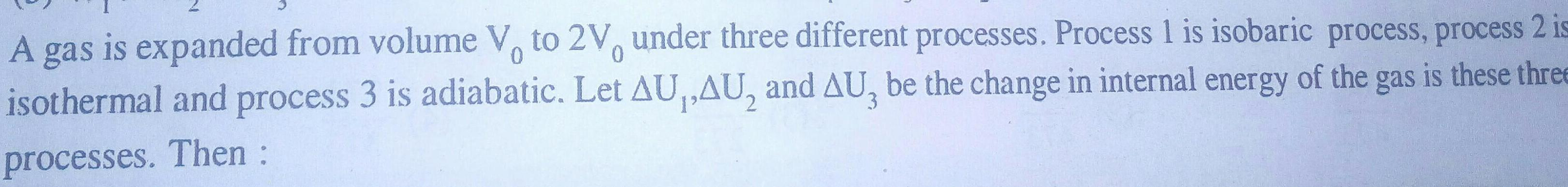Physics
Thermodynamics
A gas is expanded from volume V to 2V under three different processes Process 1 is isobaric process process 2 is 0 isothermal and process 3 is adiabatic Let AU AU and AU3 be the change in internal energy of the gas is these three processes Then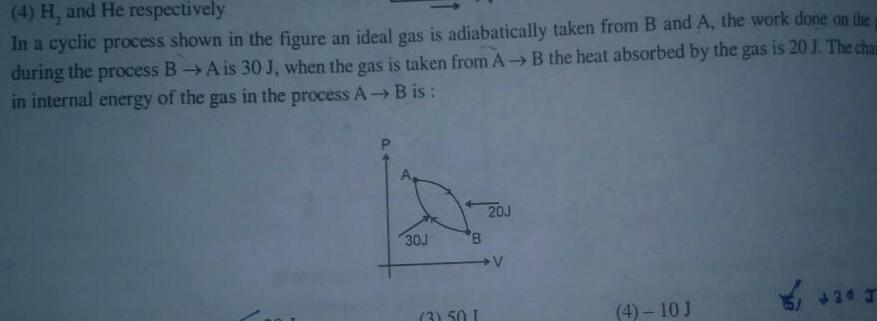Physics
Thermodynamics
4 H and He respectively In a cyclic process shown in the figure an ideal gas is adiabatically taken from B and A the work done on the p during the process B A is 30 J when the gas is taken from A B the heat absorbed by the gas is 20 J The chan in internal energy of the gas in the process A B is 30J 3 50 I 20J V 4 10 J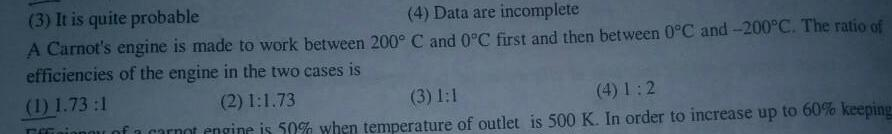Physics
Thermodynamics
3 It is quite probable 4 Data are incomplete A Carnot s engine is made to work between 200 C and 0 C first and then between 0 C and 200 C The ratio of efficiencies of the engine in the two cases is 1 1 73 1 2 1 1 73 3 1 1 4 1 2 finou of a carnot engine is 50 when temperature of outlet is 500 K In order to increase up to 60 keeping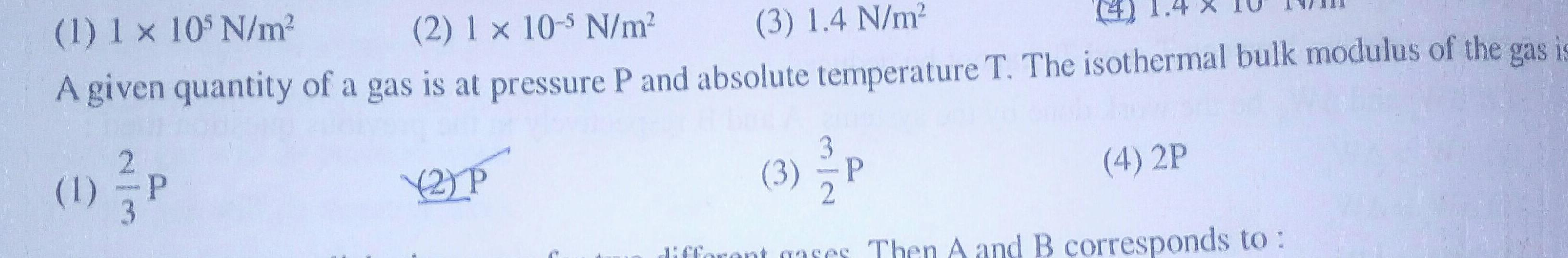Physics
Thermodynamics
1 1 x 105 N m 2 1 x 10 5 N m 3 1 4 N m A given quantity of a gas is at pressure P and absolute temperature T The isothermal bulk modulus of the gas is 1 P 2 P 3 P 4 2P Then A and B corresponds to different gases Then A and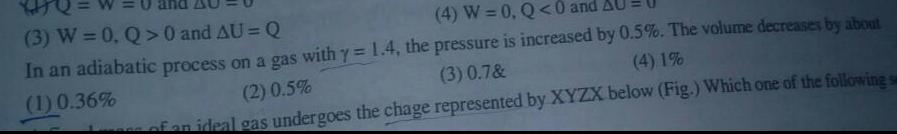Physics
Thermodynamics
3 W 0 Q 0 and AU Q 4 W 0 Q 0 and In an adiabatic process on a gas with y 1 4 the pressure is increased by 0 5 The volume decreases by about 1 0 36 2 0 5 3 0 7 4 1 of an ideal gas undergoes the chage represented by XYZX below Fig Which one of the following s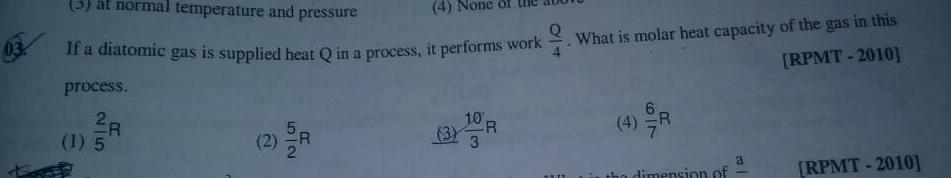Physics
Thermodynamics
3 at normal temperature and pressure 4 Q If a diatomic gas is supplied heat Q in a process it performs work process 2R 1 5 f 2 R 3 What is molar heat capacity of the gas in this RPMT 2010 dimension of a RPMT 2010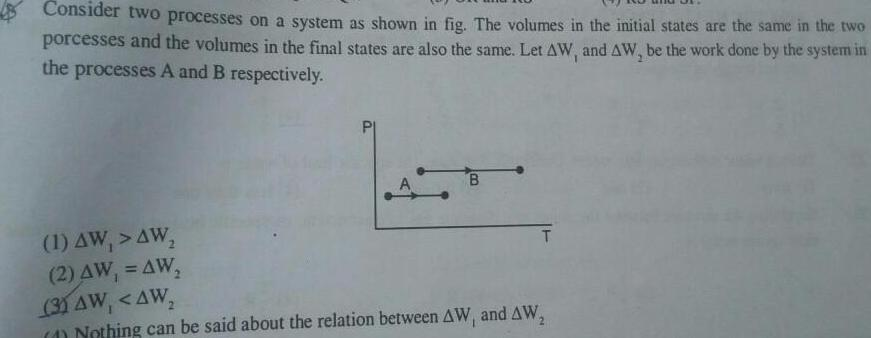Physics
Thermodynamics
Consider two processes on a system as shown in fig The volumes in the initial states are the same in the two porcesses and the volumes in the final states are also the same Let AW and AW be the work done by the system in the processes A and B respectively 1 AW AW 2 AW AW B T 3 AW AW Nothing can be said about the relation between AW and AW